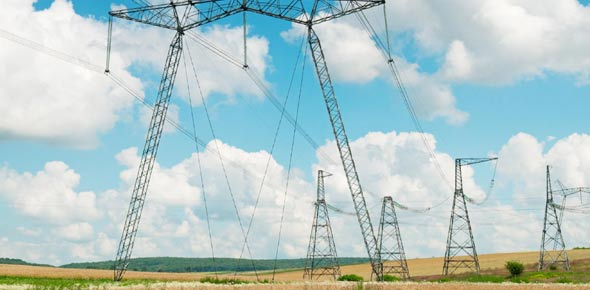10 Questions | Total Attempts: 40Settings• 1.
In 100 words, sum up the advantages and disadvantages of each type of circuit mentioned in Q4?
• 2.
What is the definition of electricity
• A.

Electricity is a form of energy which produces water

• B.

Electricity is a form of energy made available by the flow of electric charges through an electrical conductor

• C.

Electricity is lightning

• 3.
A ________ is used to open or close a circuit easily and safely.
• 4.
Electrons have _________ electric charge
• 5.
What are the two types of circuits?
• A.

Series and perpendicular

• B.

Perpendicular and Curved

• C.

Series and parallel

• D.

Parallel and Curved

• E.

Series and Curved

• 6.
What are circuit symbols used for?
• A.

Circuit symbols are used to represent each diagram for quick and easy drawing of a circuit.

• B.

Circuit symbols are used to beautify the drawing of a circuit

• C.

Circuit symbols are used to make the drawing interesting and fun for others to read

• 7.
The SI unit for electric current is ____________
• 8.
Another name to substitute the word "voltage" would be ___________
• 9.
What is the SI unit for voltage?
• A.

Volt

• B.

Volta

• C.

Tage

• D.

Watts

• E.

Milliwatt

• 10.
What are the three effects of an electric current?
• A.

Cooling, Chemical and magnetic

• B.

Heating, chemical and natural

• C.

Heating, Magnetic and natural

• D.

Cooling, Magnetic and natural

• E.

Heating, Chemical and magnetic

Related TopicsBack to top